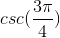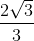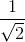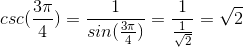Precalculus : Find the value of any of the six trigonometric functions of special angles

Example Questions

Example Question #1 : Find The Value Of Any Of The Six Trigonometric Functions Of Special Angles

In a 3-4-5 right triangle, which of the following is a possible value for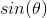?Explanation:

Sine theta is defined as the leg opposite to the angle over the hypothenuse. Write the definition of sine.  The hypotenuse is the longest side of the right triangle, which indicates that 5 should be in the denominator.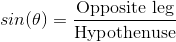The ratio of the legs opposite to theta over the hypothenuse can either beor.

Example Question #1 : Find The Value Of Any Of The Six Trigonometric Functions Of Special Angles

Find the value of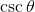.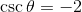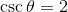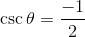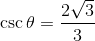Explanation:

Since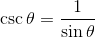we begin by finding the value of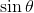.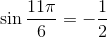.

Then,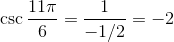Example Question #1 : Functions Of Special And Quadrantal Angles

Determine the value of: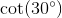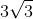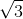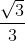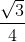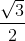Explanation:

To determine the value of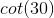, simplify cotangent into sine and cosine.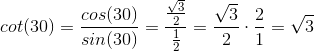Example Question #1 : Functions Of Special And Quadrantal Angles

Find the value of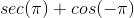, if possible.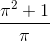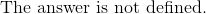Explanation:

In order to solve, split up the expression into 2 parts.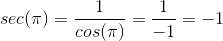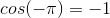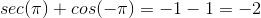Example Question #301 : Pre Calculus

Compute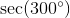, if possible.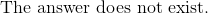Explanation:

Rewrite the expression in terms of cosine.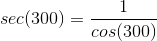Evaluate the value of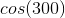, which is in the fourth quadrant.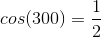Substitute it back to the simplified expression of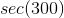.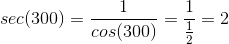Example Question #301 : Pre Calculus

Determine